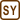## Heating of a Ball Hitting a Wooden Target

A lead ball of mass 20 g hits a massive wooden target at the speed of 100 m s−1 and it stays there. Determine the temperature increase of the ball considering that the ball absorbs two thirds of the total energy released.

• #### Hint

The kinetic energy of the ball wasn’t equal to zero before the impact. After the ball had stopped, its kinetic energy went down to zero. The assignment says that the ball absorbed two thirds of this energy.

What energy was this kinetic energy transformed to? How does it impact the properties of the ball?

• #### Analysis

Before the impact the ball had some kinetic energy. The ball stopped inside the target, hence its kinetic energy dropped to zero and transformed to internal energy of the ball and the target, which increased their temperature.

The assignment says that the ball absorbed two thirds of the energy. Due to the increase of the internal energy the temperature of the ball increased by the same amount as if the ball accepted this energy in the form of heat. With the knowledge of the energy and the specific heat capacity of lead, which can be found in Physics Reference Tables, we can determine the temperature change of the ball.

• #### Notation

 m = 20 g = 0.02 kg mass of the lead ball v = 100 ms−1 speed of the ball on impact ΔT = ? variation of temperature of the ball after impact

Found in Physics Reference Tables:

 cl = 130 J kg−1K−1 specific heat capacity of lead
• #### Solution

We calculate the initial kinetic energy Ek of the ball

$E_{\mathrm{k}}=\frac{1}{2}mv^2$

then we determine how much energy E was absorbed by the ball after impact (we know it was two thirds of the initial energy). Therefore we can write:

$E=\frac{2}{3}E_{\mathrm{k}}=\frac{2}{3}\cdot\frac{1}{2}mv^2=\frac{1}{3}mv^2.$

This energy transforms into internal energy of the ball which increases its temperature as if the ball absorbed heat Q. Now we can write the relation between the absorbed heat and the variation of temperature ΔT:

$E=Q=mc_{\mathrm{l}}\mathrm{\Delta}T$

From this relation we can evaluate ΔT and substitute for E:

$\mathrm{\Delta}T=\frac{E}{mc_{\mathrm{l}}}=\frac{mv^2}{3mc_{\mathrm{l}}}=\frac{v^2}{3c_{\mathrm{l}}}$

Note that given these conditions the temperature doesn’t depend on the mass of the ball.

Numerical insertion:

$\mathrm{\Delta}T=\frac{v^2}{3c_{\mathrm{l}}}=\frac{100^2}{3\cdot{130}}\,\mathrm{^\circ C}\dot=25.6\,\mathrm{^\circ C}$